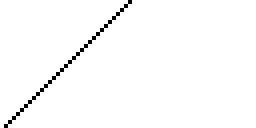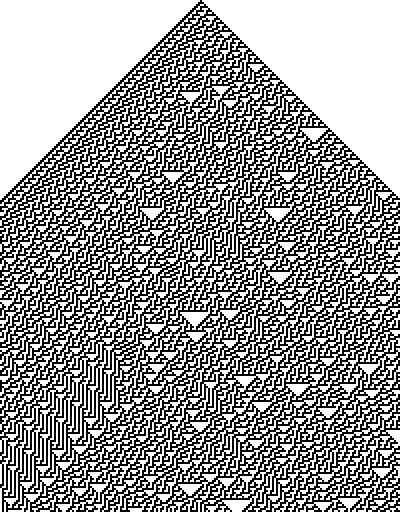Main | Generate Cellular Automata

The Cellular Automaton

The source code to my Cellular Automaton image generator can be found here. It is C++ source code that outputs a raw pgm image file to stdout. It should compile under most C++ compilers, although it has only been tested with the g++ compiler on Linux. I am releasing it under the GNU Public License (GPL), so do with it as is appropriate under that license.

 The cellular automaton consists of a line of cells, each colored either black or white. At every step there is then a definite rule that determines the color of a given cell from the color of that cell and its immediate left and right neighbors on the step before.   -Stephen Wolfram (A New Kind of Science, 2004)

Cellular automata are extreamly simple computational systems that create interesting images which show some even more interesting behaviours. Essentially, these images show the product of thousands of simple computations based on rules that should be followed regarding a pixel's imediate neighbours.

These cellular automata images are a bunch of black and white pixels that are built from the top down, where each scanline is based on the colour of the pixels on the scanline above it. More specifically, the pixels imediately above it, above it and to the left, and above it and to the right. So each pixel's colour is determined by three other pixels.

In the case of the example to the right, this rule indicates that if the pixel above and to the left is black, then the pixel in question should itself turn black.

Because each pixel can be either black or white (in a two colour cellular automaton) then this allows for two to the power of three (eight) possible colour combinations along the top three pixels. Because each of these combinations will cause a pixel to be either black or white and there are eight possible upper colour combinations then there are two to the power of eight (256) possibilities in total.

We can easily utilize a binary byte to encode these rule sets into decimal numbers between the numbers 0 and 255. In his book, A New Kind of Science, Stephen Wolfram displays the eight possible colour combinations in the following order, then indicates whether the pixel in question will be white or black with a 0 or a 1 respectively. For example, rule number 18 would correspond to the following:

0

0

0

1

0

0

1

0
= 18 (Decimal)

 (The string of eight zeros and ones create one binary byte, which can represent a decimal number between 0 and 255)

If we create a computer image where the top line is all white, and only the middle pixel is coloured black, we can then apply these rules to the pixels of each of the proceeding scanlines. When we do this, patterns emerge. Sometimes the patterns are what you would expect, simple images that follow obvious lines according to the specified rules (see rule 2 below). But amazingly detailed patterns can emerge as well (see rule 18 below).

Rule #2 Rule #18The really suprising rules are those that create complex randomness. Ones that don't follow any decernable pattern, and display an extreamly complex design such as rule 30, show below. This is interesting because the exact same process that produced the Rule #2 image was used, only a few different parameters were entered.Rule #30

To generate your own cellular automata, and experiment with the 256 different rule sets, see the cellular automaton generator.